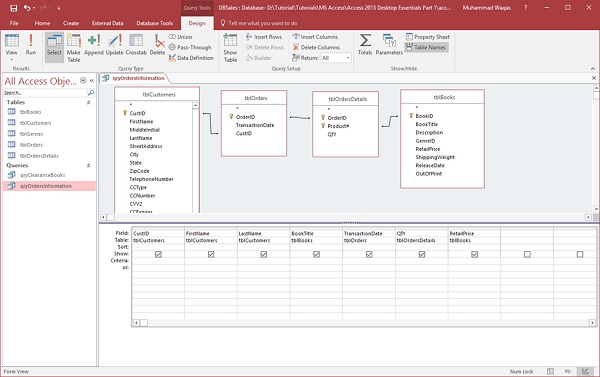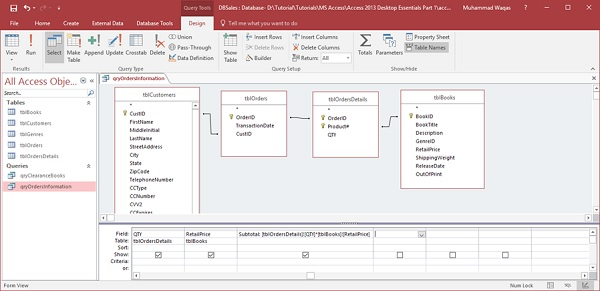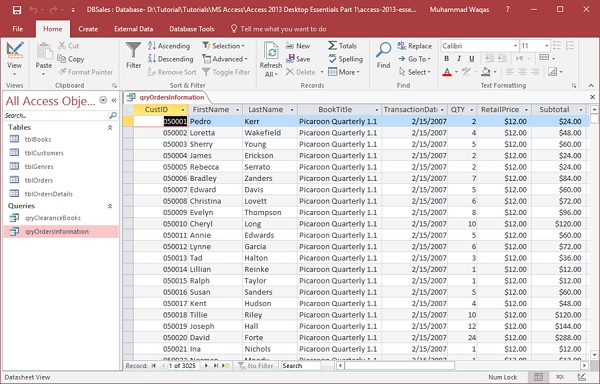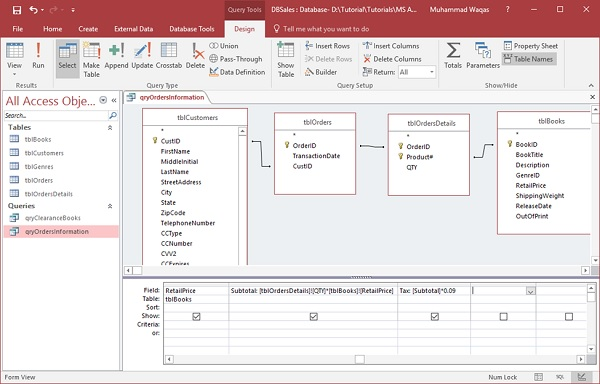# MS Access - Calculated Expression

In MS Access, an expression is like a formula in Excel. This consists of a number of elements that can be used alone or in a combination to produce a result. Expressions can include operators’, constants, functions and identifiers.

• Expressions can be used to perform a variety of tasks from retrieving the value of a control or supplying criteria to a query to creating calculated controls and fields or defining a group level for report.

• In this chapter, we will be focusing on creating a very specific kind of expression called a calculated expression. We will create several calculated fields that will calculate and display data that is not stored anywhere within the database itself but calculated from separate fields that are stored.

We now have a new Access database that contains more data to calculate.

## Example

Let us try an example to understand this. We will be retrieving some information from a variety of tables — tblCustomers, tblOrders, tblOrdersDetails and tblBooks and reviewing a variety of fields.Let us now run the query and you will see the following results.We want a very simple calculation for a subtotal which will take the quantity of the books ordered and multiply that by the retail price of the book. It is a good idea to begin every expression or any expression with a name to call that field. Let us call this one subtotal.The full expression (Subtotal: [tblOrdersDetails]![QTY]*[tblBooks]![RetailPrice]) includes the table name, first tblOrdersDetails inside square brackets because that is where our quantity field lives. Now an exclamation is telling Access to look inside that table for a field QTY and multiply that by the retail price field in tblBooks.

Let us try to run your query and you will see at end the subtotal field.Let us say we want to calculate our sales tax. In the query grid we will do in the exact same way we did before and this time we will call the field simply Tax and Let us multiply Subtotal by nine percent tax rate.Let us run your query again and you will see the new Tax field in the end.